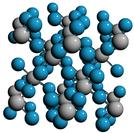Crystal Lattice Structures: Creation Date:  8 Feb 2003 Last Modified: 21 Oct 2004

# The AlF3 (D014) StructureYou can now

• Prototype: AlF3
• Pearson Symbol: hR8
• Strukturbericht Designation: D014
• Space Group: R32 (Cartesian and lattice coordinate listings available)
• Number: 155
• Other Compounds with this Structure: FeF3
• Primitive Vectors:

The rhombohedral lattice can be specified by the length of the primitive vectors, a, and the angle between them, θ, or by the lattice constants of the equivalent hexagonal lattice. In terms of a and θ we have

ahex = 2 a sin(½ θ)

chex = a {3 [1 + 2 cos(θ)]}½

In rhombohedral form:

 A1 = + ½ ahex X - 12-½ ahex Y + 1/3 chex Z A2 = + 3-½ ahex Y + 1/3 chex Z A3 = - ½ ahex X - 12-½ ahex Y + 1/3 chex Z
• Basis Vectors:  B1 = + z A1 + z A2 + z A3 = + z c Z (F) (2c) B2 = - z A1 - z A2 - z A3 = - z c Z (F) (2c) B3 = + y1 A2 - y1 A3 = ½ y1 a X + ½ 3½ y1 a Y (F-I) (3d) B4 = - y1 A1 + y1 A3 = - y1 a X (F-I) (3d) B5 = + y1 A1 - y1 A2 = ½ y1 a X - ½ 3½ y1 a Y (F-I) (3d) B6 = ½ A1 + y2 A2 - y2 A3 = ¼ (2 y2 + 1) a X + 48-½ (6 y2 - 1) a Y + 1/6 c Z (F-II) (3e) B7 = - y2 A1 + ½ A2 + y2 A3 = - y2 a X + 12-½ a Y + 1/6 c Z (F-II) (3e) B8 = + y2 A1 - y2 A2 + ½ A3 = ¼ (2 y2 - 1) a X - 48-½ (6 y2 + 1) a Y + 1/6 c Z (F-II) (3e)

Go back to the IIIb-VIIb compounds page.

Go back to Crystal Lattice Structure page.

 Structures indexed by: This is a mirror of an old page created at theNaval Research LaboratoryCenter for Computational Materials ScienceThe maintained successor is hosted at http://www.aflowlib.org/CrystalDatabase/ and published as M. Mehl et al., Comput. Mater. Sci. 136 (Supp.), S1-S828 (2017).